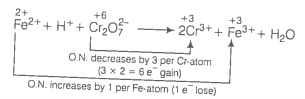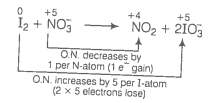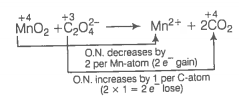24. Balance the following equations by the oxidation number method.

(a) ${\mathrm{Fe}}^{2+}+{\mathrm{H}}^{+}+{\mathrm{Cr}}_{2}{\mathrm{O}}_{7}^{-2}\to {\mathrm{Cr}}^{2+}+{\mathrm{Fe}}^{3+}+{\mathrm{H}}_{2}\mathrm{O}$

(b) ${\mathrm{I}}_{2}+{\mathrm{NO}}_{3}^{-}\to {\mathrm{NO}}_{2}+{\mathrm{IO}}_{3}^{-}$

(c) ${\mathrm{I}}_{2}+{\mathrm{S}}_{2}{\mathrm{O}}_{3}^{2-}\to {\mathrm{I}}^{-}+{\mathrm{S}}_{4}{\mathrm{O}}_{6}^{2-}$

(d) ${\mathrm{MnO}}_{2}+{\mathrm{C}}_{2}{\mathrm{O}}_{4}^{2-}\to {\mathrm{Mn}}^{2+}+{\mathrm{CO}}_{2}$

Oxidation number method
(a)(Multiply Cr3+ by 2 because there are 2Cr atoms in ${\mathrm{Cr}}_{2}{\mathrm{O}}_{7}^{2-}$ ion.)
Balance increase and decrease in oxidation number.
$6{\mathrm{Fe}}^{2+}+{\mathrm{H}}^{+}+{\mathrm{Cr}}_{2}{\mathrm{O}}_{7}^{-2}\to 2{\mathrm{Cr}}^{2+}+6{\mathrm{Fe}}^{3+}+{\mathrm{H}}_{2}\mathrm{O}$
Balance charge by multiplying H+ by 14.
$6{\mathrm{Fe}}^{2+}+14{\mathrm{H}}^{+}+{\mathrm{Cr}}_{2}{\mathrm{O}}_{7}^{-2}\to 2{\mathrm{Cr}}^{2+}+6{\mathrm{Fe}}^{3+}+{\mathrm{H}}_{2}\mathrm{O}$
Balance H and O-atoms by multiplying H2O by 7.
$6{\mathrm{Fe}}^{2+}+14{\mathrm{H}}^{+}+{\mathrm{Cr}}_{2}{\mathrm{O}}_{7}^{-2}\to 2{\mathrm{Cr}}^{2+}+6{\mathrm{Fe}}^{3+}+7{\mathrm{H}}_{2}\mathrm{O}$
This represents a balanced redox reaction.
(b)(Multiply ${\mathrm{S}}_{2}{\mathrm{O}}_{3}^{2-}$ by 2 because there are 4 S-atoms in ${\mathrm{S}}_{4}{\mathrm{O}}_{6}^{2-}$ ion.)
Increase and decrease in oxidation number is already balanced. Charge and oxygen atoms are also balanced.
This represents a balanced redox reaction.
(c)Increase and decrease in oxidation number is already balanced.
Add 4H+ towards LHS of the equation to balance charge.
${\mathrm{MnO}}_{2}+{\mathrm{C}}_{2}{\mathrm{O}}_{4}^{2-}+4{\mathrm{H}}^{+}\to {\mathrm{Mn}}^{2+}+2{\mathrm{CO}}_{2}$
Add 2H2O towards RHS of the equation to balance H-atoms.
${\mathrm{MnO}}_{2}+{\mathrm{C}}_{2}{\mathrm{O}}_{4}^{2-}+4{\mathrm{H}}^{+}\to {\mathrm{Mn}}^{2+}+2{\mathrm{CO}}_{2}+2{\mathrm{H}}_{2}\mathrm{O}$
This represents a balanced redox reaction.